2022: SklogWiki celebrates 15 years on-line

# Helmholtz energy function

Helmholtz energy function (Hermann Ludwig Ferdinand von Helmholtz) Definition of$A$ (for arbeit):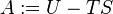$A:=U-TS$

where U is the internal energy, T is the temperature and S is the entropy. (TS) is a conjugate pair. The differential of this function is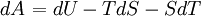$\left.dA\right.=dU-TdS-SdT$

From the second law of thermodynamics one obtains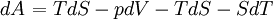$\left.dA\right.=TdS -pdV -TdS-SdT$

thus one arrives at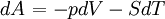$\left.dA\right.=-pdV-SdT$.

For A(T,V) one has the following total differential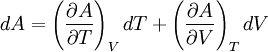$dA=\left(\frac{\partial A}{\partial T}\right)_V dT + \left(\frac{\partial A}{\partial V}\right)_T dV$

The following equation provides a link between classical thermodynamics and statistical mechanics: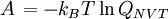$\left.A\right.=-k_B T \ln Q_{NVT}$

where$k_B$ is the Boltzmann constant, T is the temperature, and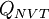$Q_{NVT}$ is the canonical ensemble partition function.

## Ideal gas

Main article: Ideal gas Helmholtz energy function

## Quantum correction

A quantum correction can be calculated by making use of the Wigner-Kirkwood expansion of the partition function, resulting in (Eq. 3.5 in ):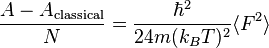$\frac{A-A_{ {\mathrm{classical}} }}{N} = \frac{\hbar^2}{24m(k_BT)^2} \langle F^2 \rangle$

where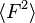$\langle F^2 \rangle$ is the mean squared force on any one atom due to all the other atoms.